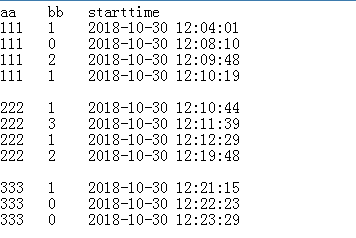mysql统计某字段值相同情况时，对应另外一个字段值变化次数（已补充时间条件排序） 5C1则统计值加1

111 为 2
222 为 3
333 为 1

aa里面出现的值我是未知的 ，求解select 语句 该怎么写

5个回答

333 1
333 0
333 0

333 0
333 1
333 0qian_yan_ai 大佬求解啊 我没说清楚，是有一个时间字段可以用来排序的
11 个月之前 回复qian_yan_ai 回复: 假如有一个starttime的字段表示先后呢？
11 个月之前 回复qian_yan_ai 里面有一个时间的字段，是按照时间先后的
11 个月之前 回复

aa字段的值和统计结果可以用字典存储

``````select
case when @b<>aa then @a:=-1 end,
aa,
@a,
case when @a<>1 and bb=1 and @a<>-1 then 1
when @a=1 and bb<>1 then 1 end as if_valid,
@a:=bb,
@b:=aa
from (select @a:=-1) a,
(select @b:=-1)c,
(select * from xx order by aa,starttime asc) b
;
``````

select gy,count(if_valid) from (
select
case when @b<>aa then @a:=-1 end,
aa,
@a,
case when @a<>1 and bb=1 and @a<>-1 then 1
when @a=1 and bb<>1 then 1 end as if_valid,
@a:=bb,
@b:=aa as gy
from (select @a:=-1) a,
(select @b:=-1)c,
(select * from xx order by aa,starttime asc) b ) yy group by gy
;